Volume 4 Supplement 1

# A statistical method for adjusting covariates in linkage analysis with sib pairs

## Abstract

### Background

We propose a statistical method that includes the use of longitudinal regression models and estimation procedures for adjusting for covariate effects in applying the Haseman-Elston (HE) method for linkage analysis. Our methodology, which uses the covariate adjusted trait, contains three steps: a) modelling the covariate-adjusted population means of quantitative traits through regression; b) estimating the value of covariate-adjusted quantitative traits; and c) evaluating the linkage between the adjusted trait values and the markers based on alleles shared identically by descent.

### Results

We applied our adjusted HE method and the standard HE method in S.A.G.E. to the sib-pair subset of the Framingham Heart Study distributed by Genetic Analysis Workshop 13 with systolic blood pressure as the quantitative trait. Both methods gave similar patterns for the LOD scores, and exhibited highest multipoint LOD scores near location 70 cM of chromosome 12.

### Conclusion

The adjusted HE method has two major advantages over the standard HE method used in S.A.G.E.: a) it has the capability to handle longitudinal data; b) it provides a more natural approach for adjusting the repeatedly measured covariates from each subject.

## Background

Let X1jand X2jbe the observed trait values for the first and second sibs in a cross-sectional study, and let Y j = (X1j- X2j)2 be the squared trait difference in the jth sib pair. The Haseman-Elston (HE) method  assumes that

X ij = μ + g ij + e ij ,     (1)

where i = 1,2, μ is the overall mean trait value, and g ij and e ij , which are independent and have mean zero, represent the genetic and environmental effects, respectively, on the ith sib of the jth sib pair [denoted by the (i, j)th sib hereafter].

Suppose that, in addition to the genetic and environmental effects, the phenotype X ij is also affected by a set of p covariates. Let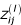be the value observed for the lth covariate in the (i, j)th sib (j = 1,2; i = 1,...,n; l = 1,...,p). Given the proportion of genes identical by decent (IBD), π j for the jth sib pair and covariatesfor the (i, j)th sib, Elston et al.  described the genetic and covariate effects on the phenotype through the linear modelwhere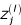is a transformed covariate determined byand, β0 describes the linkage between the phenotype and the marker alleles, and β1,...,β p represents the covariate effects. Estimation and inference procedures based on equation (2) have been incorporated into the software package SIBPAL in S.A.G.E. .

In practice, however, there are two potential limitations for the method based on equation (2). First, since the model and its estimation procedures currently used in S.A.G.E.  are designed only for cross-sectional studies, they are generally not suitable for longitudinal studies where the data are repeatedly obtained over time. Second, in some situations it may not be appropriate to selectas the original covariates observed in the data set, so that an adequate implementation of equation (2) depends on choosing a sensible transformation applied to the original covariates. To overcome these shortcomings, we propose in this paper an alternative sib-pair approach that can be applied to longitudinal studies and that adjusts the covariates prior to linkage analysis. The approach of adjusting covariate prior to linkage analysis has been previously considered in the literature under some different contexts (e.g., Amos  and Suh et al. ). Our method is focused on combining the HE method with statistical procedures for covariate adjustment using the generalized estimating equations (GEE) and within-cluster resampling  and contains three main steps: a) modelling the covariate-adjusted population means of quantitative traits through regression; b) estimating the covariate-adjusted quantitative traits; and c) evaluating the linkage between the adjusted trait values and the marker alleles shared IBD. The objective is to link phenotype with the proportion of genes shared IBD using only the trait values after removing the influences of the covariates that are unrelated to the genes. Numerical computations of our method can be easily implemented using the existing statistical and genetic software packages, such as SAS (SAS/STAT Software ) and SIBPAL (S.A.G.E. ).

Applying our method and the standard HE method (equation (2)) in S.A.G.E. to the Genetic Analysis Workshop 13 Framingham Heart Study data, we found that both methods gave similar results for cross-sectional analyses using only the data from one visit, and exhibited highest multipoint LOD scores near location 70 cM of chromosome 12 for the longitudinal analyses. Our method is more natural at handling the longitudinal data and has generally higher peak multipoint LOD scores than the standard HE method.

## Methods

### Modelling the covariates

For cross-sectional studies, we assume that the covariates are not affected by the genes and generalize equation (1) towhereis the expectation of X ij given the covariatesand the unknown (p + 1) dimensional parameter ψ, andis the covariate adjusted trait for the (i, j)th sib. When μ(·;·) is a simple linear link function, the value of the covariate adjusted phenotype is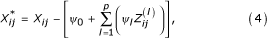where ψ = (ψ0,...,ψ p ) are the linear coefficients.

For longitudinal studies, let n ij be the number of repeated measurements and T ijk , k = 1,...,n ij , be the time of the kth measurement for the (i, j)th sib. Assume that the conditional expectation of X ijk given the covariate vector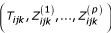is, which is determined by the (p + 2) dimensional parameter ψ and holds for all the measurement points. Assume further that the gene effects on the quantitative trait values are the same for all the measurement points. By adjusting the time-dependent covariates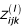, we have the longitudinal modelThen the linear model with coefficients ψ0,...,ψp+1 is### Methods for cross-sectional studies

Using the covariate adjusted squared trait difference, the same derivation as in Table 1 of HE  shows that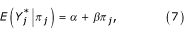and the problem of linkage detection reduces to testing the statistical hypothesis of β = 0 (no linkage) versus the one-sided alternative β < 0 (linkage). If there were a consistent estimate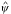for ψ, thenandcan be estimated byand, respectively. The hypotheses β = 0 and β < 0 can be tested using the standard HE procedure withas the observed squared trait difference.

Since the sibs in the same family are correlated, there are two approaches that can be used to estimate ψ. The first is to treat the families as independent subjects and apply the existing longitudinal methods, such as the generalized estimating equations (GEE, e.g., [6, 10]) to the observed traits. The second approach is within-cluster resampling [7, 8], which first generates multiple independent resampled data sets by randomly drawing one sib from each family, estimates ψ from each resampled data set using the existing estimation methods for independent cross-sectional data, and finally computesby averaging the estimates computed from all the resampled data. When the number of families is large, both approaches are expected to lead to consistent estimates.

### Methods for longitudinal studies

We assume here that both sibs in a pair have the same number of repeated measurements, i.e., n1j= n2j= n j for all j. The adjusted squared trait difference at the kth measurement for the jth pair is, and averaging over all the measurements, the average adjusted squared trait difference for the jth pair is. Then equation (7) continues to hold ifwere replaced by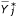, and, consequently, the linkage between the phenotype and the marker loci can be detected by testing β = 0 against β < 0. If there were a consistent estimate, we could estimateby, whereand the standard HE procedure would be implemented usingas the observed squared trait difference for the jth pair.

The estimation of ψ is now affected by two sources of correlations: the correlation between the repeated measurements within a sib and the correlations between sibs within the same family. Ideally, it is possible to model these correlation structures and incorporate these correlation models into an established longitudinal estimation procedure, such as GEEs. But the potential bias and variability that may be associated with the possible model misspecifications of this approach have not been well studied. As a practical alternative, we suggest the following three-step within-cluster resampling procedure: a) randomly sample one sib from each family; b) estimate ψ from the resampled data using GEE; and c) repeat the above steps multiple times and calculateby averaging the estimates from all the resampled data sets. When the number of families is large,is expected to be a consistent estimate of ψ [7, 8].

### Choosing correlation structures in GEE

An important issue in obtaining an appropriate value for a covariate-adjusted phenotype is to select a suitable covariate structure for implementing the GEE procedure in SAS or other statistical programs. For cross-sectional studies, equation (3) is equivalent towhereis the marginal mean of X ij givenand ψ, and ε ij is the error term determined by the gene and the environment. This model falls into the framework of marginal models of Diggle et al. [, Ch. 7]. When GEE is used for estimating the unknown parameter ψ of the marginal component, a suitable covariance structure that can be generally applied with the PROC MIXED procedure in SAS  is the "compound symmetry" model. Explicit mathematical expression of the compound symmetric covariance structure is given by Verbeke and Molenberghs [, page 117]. Other covariance structures such as the ones described in Diggle et al. [, Ch. 5] may also be considered when additional details about the error term ε ij are available.

For longitudinal studies, we can apply the GEE procedure with the same compound symmetric covariance structure as in Verbeke and Molenberghs [, page 117] to the within-cluster resampled data with the marginal modelso that consistent estimatefor ψ in the marginal component can be obtained. Since only one sib in a family is randomly chosen in the resampled data, compound symmetry is an adequate assumption for the correlation structure here.

### Comparison with the standard HE method

Theoretically, our covariate-adjusted linear model (equation (7)) is equivalent to the standard HE model (equation (2)) if proper transformations for the original covariates are used. To see this, we compare these models for the cross-sectional data, as similar arguments can be made for the case with longitudinal data. Let μ ij =. Assume, for simplicity, that the covariatesare non-random. (The same conclusion for the random covariate case can be similarly derived by taking conditional expectations given these covariates, assuming thatare independent of π j .) Direct calculation using the definitions of Y j andthen shows that

E(| π j ) = E(Y j | π j ) - (μ1j- μ2j)2 = α + βπ j .     (8)

When μ ij is a simple linear link function as given in the second term at the right side of the equation (4), equation (8) is clearly a special form of equation (2) with the covariates at the right side of equation (2) taken to be quadratic and cross-product transformations of the original covariates. When μ ij is a nonlinear function of the original covariates, suitable covariate transformations have to be used to make equation (2) equivalent to equation (8). In practice, however, it is often difficult to determine what meaningful covariate transformation should be used in equation (2). In our view, the appeal of equation (7) or its equivalent, equation (8), compared to the standard HE method is that the variations on phenotype contributed by the nuisance factors (covariates unaffected by genes) can be naturally modelled and adjusted before analysing the gene effects.

## Data

The second generation data from the Framingham Heart Study distributed by the Genetic Analysis Workshop 13 contain 482 multi-sib families from a total of 576 nuclear families from 330 pedigrees. We used all the possible sib pairs from these families and the repeated measurements from all their visits. For the purpose of illustration, we specified systolic blood pressure (SBP) as the quantitative trait, and the subject's age (in years), gender (0 for female, and 1 for male), and average daily alcohol consumption (in milliliters) as the covariates of interest. The subjects had up to five visits during the study, but not all the subjects completed all five visits. Among a total of 1672 subjects that were included in the data set, the numbers of subject who were measured at visits 1 through 5 were 1649, 1393, 1402, 1439, and 1377, respectively.

## Implementation

We assumed that the mean SBP conditioning on the subject's gender, and age and drinking level (ml/day) at the visit was determined by

μ (gender, age, drink; ψ) = ψ0 + ψ1 × gender + ψ2 × age + ψ3 × drink.

Using the three-step resampling procedure, we generated 1000 independent resampled data sets from the entire sib-pair data that included all the observed visits, and computedby averaging the GEE estimates obtained from the resampled data sets using the compound symmetric covariance structure. For each sib pair, we used the visits in which the measurements were available for both sibs, estimated the covariate adjusted SBP at the kth visit byand computed the adjusted squared SBP difference bywhere n j is the number of common visits for both sibs of the jth sib pair. We then performed the genome scan using the adjusted squared SBP difference and the existing HE procedure in S.A.G.E. .

For comparison, we performed the cross-sectional analysis using the adjusted HE method as above on data from visit 1. This visit was used because it had the measurements for most of the participating subjects. Since only one visit was used, the phenotype for each sib pair was simply the squared difference of the sibs' covariate adjusted SBP.

### Standard HE method

The current HE procedure in S.A.G.E. is not capable of handling the longitudinal data from multiple visits. In order to transform the measurements from multiple visits into the data structure that can be taken by S.A.G.E., we used for each subject the average values of his/her SBP, age, and drinking level over his/her available visits, and fitted the model (2) using the phenotype

Y j = [(average SBP)1j- (average SBP)2j]2

and the covariatesandwhere (average A) ij denotes the averaged value of A for the (i, j)th sib over his/her repeated measurements.

Although the above approach is ad hoc, it is a practical way for transforming repeated measurements to the data framework currently acceptable by S.A.G.E., and should be generally acceptable if the data are balanced in the sense that there are very few missing values in the data set. However, when the data are unbalanced, the approach of averaging out observed measurements over different time points may lead to potential bias for the analysis. One approach for handling the imbalance caused by missing data is to combine the above approach with multiple imputation. But its adequacy under the current setting deserves further investigation.

To compare the standard HE method with our adjusted HE method in cross-sectional data, we fitted the standard HE model using only the data from visit 1 with the squared trait difference Y j = (SBP1j-SBP2j)2, and covariatesThe same procedure can be repeated for cross-sectional data from other visits. But, since the results from the cross-sectional analyses do not dramatically differ from one another, we only included the results for visit 1 in the presentation.

## Results

### Longitudinal analyses

Figure 1 shows the multipoint LOD scores based on the adjusted HE method and the standard HE method for all 22 chromosomes. Both methods exhibit similar general patterns for the LOD scores. The largest LOD scores are 2.3 to 2.6 for the adjusted HE method and the standard HE method, respectively, both appear near position 70 cM of chromosome 12. The adjusted HE method also shows a few higher LOD score peaks than the standard HE method at other locations. But all these LOD scores are smaller than 1.6.

### Cross-sectional analyses

Figure 2 shows the multipoint LOD scores for all 22 chromosomes based on the visit 1 data. The LOD scores under both methods have similar patterns. Compared with the longitudinal analysis, the LOD score peaks at chromosome 12 are slightly smaller and their locations are slightly shifted; however, several peaks, such as the ones in chromosomes 4 and 11, do not appear in the longitudinal analyses.

## Discussion

We have proposed a new statistical method for adjusting the effects of covariates for the HE regression when longitudinal data are present. Compared with the linear covariate adjustment in S.A.G.E., our method has two advantages. First, it can handle repeated measurements in a longitudinal study using the existing software such as SAS and S.A.G.E. Second, it adjusts the covariates prior to linkage analysis in a natural way. There are a number of possibilities to generalize our approach. One option is to replace the additive assumptions on the gene and environmental effects in equation (5) by terms that allow for interactions. Other options may aim at modelling and estimating the covariate effects based on more general parametric and nonparametric statistical models. These generalizations require redeveloping the relationship between the trait difference and the proportion of genes IBD.

## References

1. 1.

Haseman JK, Elston RC: The investigation of linkage between a quantitative trait and a marker locus. Behav Genet. 1972, 2: 3-19. 10.1007/BF01066731.

2. 2.

Elston RC, Buxbaum SJ, Jacobs KB, Olson JM: Haseman and Elston revisited. Genetic Epidemiology. 2000, 19: 1-17. 10.1002/1098-2272(200007)19:1<1::AID-GEPI1>3.0.CO;2-E.

3. 3.

Case Western Reserve University: S.A.G.E. Statistical Analysis for Genetic Epidemiology, Beta 4.0-7. Department of Epidemiology and Biostatistics, Rammelkamp Center for Education and Research, MetroHealth Campus, Cleveland, Ohio, Case Western Reserve University. 2002

4. 4.

Amos AI: Robust variance-components approach for assessing genetic linkage in pedigrees. Am J Hum Genet. 1994, 54: 535-543.

5. 5.

Suh YJ, Finch SJ, Mendell NR: Application of a Bayesian method for optimal subset regression to linkage analysis of Q1 and Q2. Genet Epidemiol. 2001, 21 (suppl 1): S706-S711.

6. 6.

Diggle PJ, Liang KY, Zeger SL: Analysis of Longitudinal Data. Oxford, UK, Oxford University Press. 1994

7. 7.

Follmann D, Proschan MA, Leifer E: Multiple outputation: inference for complex multivariate data by averaging analyses from univariate data. Biometrics. 2003, 53: 420-429. 10.1111/1541-0420.00049.

8. 8.

Hoffman EB, Sen PK, Weinberg C: Within-cluster resampling. Biometrika. 2001, 88: 1121-1134. 10.1093/biomet/88.4.1121.

9. 9.

SAS Institute Inc: SAS/STAT Software: Changes and Enhancements Through Release 6.12. Cary, NC, SAS Institute Inc. 1997

10. 10.

Verbeke G, Molenberghs E: Linear Mixed Models for Longitudinal Data. New York, Springer. 2000

## Acknowledgments

We thank Dr. Mario Stylianou for his help on producing the graphs. The results reported were obtained by using the program package S.A.G.E., which is supported by U.S. Public Health Service Resource grant RR03655 from the National Center for Research Resources.

## Author information

Authors

### Corresponding author

Correspondence to Colin O Wu.

## Rights and permissions

Reprints and Permissions

Wu, C.O., Zheng, G., Leifer, E. et al. A statistical method for adjusting covariates in linkage analysis with sib pairs. BMC Genet 4, S51 (2003). https://doi.org/10.1186/1471-2156-4-S1-S51